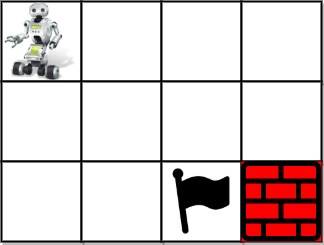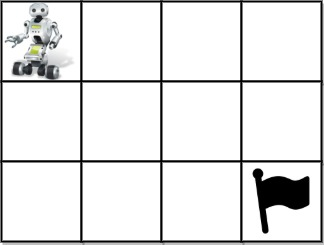## Algorithm

Problem Name: 980. Unique Paths III

You are given an `m x n` integer array `grid` where `grid[i][j]` could be:

• `1` representing the starting square. There is exactly one starting square.
• `2` representing the ending square. There is exactly one ending square.
• `0` representing empty squares we can walk over.
• `-1` representing obstacles that we cannot walk over.

Return the number of 4-directional walks from the starting square to the ending square, that walk over every non-obstacle square exactly once.

Example 1:```Input: grid = [[1,0,0,0],[0,0,0,0],[0,0,2,-1]]
Output: 2
Explanation: We have the following two paths:
1. (0,0),(0,1),(0,2),(0,3),(1,3),(1,2),(1,1),(1,0),(2,0),(2,1),(2,2)
2. (0,0),(1,0),(2,0),(2,1),(1,1),(0,1),(0,2),(0,3),(1,3),(1,2),(2,2)
```

Example 2:```Input: grid = [[1,0,0,0],[0,0,0,0],[0,0,0,2]]
Output: 4
Explanation: We have the following four paths:
1. (0,0),(0,1),(0,2),(0,3),(1,3),(1,2),(1,1),(1,0),(2,0),(2,1),(2,2),(2,3)
2. (0,0),(0,1),(1,1),(1,0),(2,0),(2,1),(2,2),(1,2),(0,2),(0,3),(1,3),(2,3)
3. (0,0),(1,0),(2,0),(2,1),(2,2),(1,2),(1,1),(0,1),(0,2),(0,3),(1,3),(2,3)
4. (0,0),(1,0),(2,0),(2,1),(1,1),(0,1),(0,2),(0,3),(1,3),(1,2),(2,2),(2,3)
```

Example 3:```Input: grid = [[0,1],[2,0]]
Output: 0
Explanation: There is no path that walks over every empty square exactly once.
Note that the starting and ending square can be anywhere in the grid.
```

Constraints:

• `m == grid.length`
• `n == grid[i].length`
• `1 <= m, n <= 20`
• `1 <= m * n <= 20`
• `-1 <= grid[i][j] <= 2`
• There is exactly one starting cell and one ending cell.

## Code Examples

### #1 Code Example with Java Programming

```Code - Java Programming```

``````
class Solution {
int[][] dirs = {{1, 0}, {0, 1}, {-1, 0}, {0, -1}};
public int uniquePathsIII(int[][] grid) {
if (grid.length == 0 || grid.length == 0) {
return 0;
}
int rows = grid.length;
int cols = grid.length;
int startX = 0;
int startY = 0;
int numOfEmptySquare = 0;
for (int i = 0; i < rows; i++) {
for (int j = 0; j < cols; j++) {
if (grid[i][j] == 1) {
startX = i;
startY = j;
}
if (grid[i][j] == 0) {
numOfEmptySquare++;
}
}
}
int[] count = {0};
helper(grid, startX, startY, numOfEmptySquare, 0, new boolean[rows][cols], count);
return count;
}

private void helper(
int[][] grid, int startX, int startY, int numOfEmptySquare,
int currEmptySquare, boolean[][] visited, int[] count
) {
if (startX < 0 || startX >= grid.length || startY < 0 || startY >= grid.length || grid[startX][startY] == -1 || visited[startX][startY]) {
return;
}
if (grid[startX][startY] == 2) {
if (currEmptySquare == numOfEmptySquare) {
count++;
}
return;
}
visited[startX][startY] = true;
for (int[] dir : dirs) {
helper(grid, startX + dir, startY + dir, numOfEmptySquare, (grid[startX][startY] == 0 ? currEmptySquare + 1 : currEmptySquare), visited, count);
}
visited[startX][startY] = false;
}
}

``````
Copy The Code &

Input

cmd
grid = [[1,0,0,0],[0,0,0,0],[0,0,2,-1]]

Output

cmd
2

### #2 Code Example with Javascript Programming

```Code - Javascript Programming```

``````
const uniquePathsIII = function(grid) {
const obj = {
eNum: 1,
res: 0,
rows: grid.length,
dirs: [[-1, 0], [1, 0], [0, -1], [0, 1]],
sr: 0,
sc: 0,
er: 0,
ec: 0
}
if (obj.rows === 0) return 0
obj.cols = grid.length
for (let i = 0; i < obj.rows; i++) {
for (let j = 0; j < obj.cols; j++) {
if (grid[i][j] === 0) obj.eNum++
else if (grid[i][j] === 1) (obj.sr = i), (obj.sc = j)
else if (grid[i][j] === 2) (obj.er = i), (obj.ec = j)
}
}
bt(grid, obj.sr, obj.sc, obj)
return obj.res
}

function bt(grid, x, y, obj) {
if (x < 0 || x >= obj.rows || y < 0 || y >= obj.cols || grid[x][y] < 0) return
if (x === obj.er && y === obj.ec) {
if (obj.eNum === 0) obj.res++
return
}
grid[x][y] = -2
obj.eNum--
for (let dir of obj.dirs) {
bt(grid, x + dir, y + dir, obj)
}
obj.eNum++
grid[x][y] = 0
}
``````
Copy The Code &

Input

cmd
grid = [[1,0,0,0],[0,0,0,0],[0,0,2,-1]]

Output

cmd
2

### #3 Code Example with Python Programming

```Code - Python Programming```

``````
class Solution:
def uniquePathsIII(self, grid: List[List[int]]) -> int:
def dfs(i, j, visited):
if grid[i][j] == 2:
self.walks += visited == self.visit
return
for x, y in ((i - 1, j), (i + 1, j), (i, j - 1), (i, j + 1)):
if 0 <= x < m and 0 <= y < n and grid[x][y] != -1:
grid[i][j] -= 1
dfs(x, y, visited + 1)
grid[i][j] += 1
m, n = len(grid), len(grid)
self.visit = m * n - sum(c == -1 for row in grid for c in row)
self.walks = 0
for i in range(m):
for j in range(n):
if grid[i][j] == 1:
grid[i][j] -= 1
dfs(i, j, 1)
return self.walks
``````
Copy The Code &

Input

cmd
grid = [[1,0,0,0],[0,0,0,0],[0,0,0,2]]

Output

cmd
4

### #4 Code Example with C# Programming

```Code - C# Programming```

``````
namespace LeetCode
{
public class _0980_UniquePathsIII
{
private readonly (int dr, int dc)[] direction = new (int dr, int dc) { (1, 0), (-1, 0), (0, 1), (0, -1) };
private int count = 0;

public int UniquePathsIII(int[][] grid)
{
int rows = grid.Length, cols = grid.Length;
int nonObstacles = 0, startRow = -1, startCol = -1;

for (int row = 0; row < rows; row++)
for (int col = 0; col < cols; col++)
{
int cell = grid[row][col];
if (cell >= 0)
nonObstacles += 1;
if (cell == 1)
{
startRow = row;
startCol = col;
}
}

Backtrack(grid, startRow, startCol, nonObstacles);
return this.count;
}

private void Backtrack(int[][] grid, int row, int col, int nonObstacles)
{
if (grid[row][col] == 2 && nonObstacles == 1)
{
this.count++;
return;
}

int rows = grid.Length, cols = grid.Length;
int temp = grid[row][col];
grid[row][col] = -2;
nonObstacles -= 1;

foreach ((int dr, int dc) in direction)
{
int newRow = row + dr;
int newCol = col + dc;

if (newRow >= 0 && newRow < rows && newCol >= 0 && newCol < cols && grid[newRow][newCol] >= 0)
Backtrack(grid, newRow, newCol, nonObstacles);
}

grid[row][col] = temp;
}
}
}
``````
Copy The Code &

Input

cmd
grid = [[1,0,0,0],[0,0,0,0],[0,0,0,2]]

Output

cmd
4